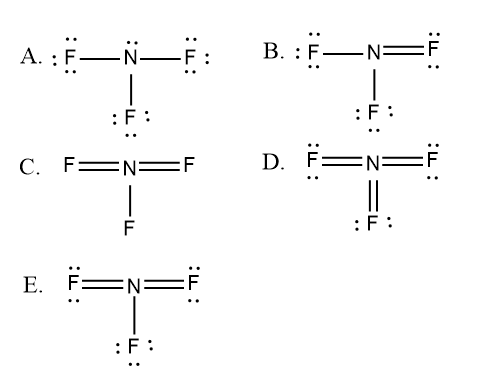Problem: Select the correct Lewis structure for nitrogen trifluoride.

100% (5 ratings)
FREE Expert Solution

We’re being asked to figure out the correct Lewis structure for NF3.

To do so, we first need to do the following steps:

Step 1: Determine the central atom in this molecule.

Step 2: Calculate the total number of valence electrons present.

Step 3: Draw the Lewis structure for the molecule.

100% (5 ratings)
Problem Details

Select the correct Lewis structure for nitrogen trifluoride.What scientific concept do you need to know in order to solve this problem?

Our tutors have indicated that to solve this problem you will need to apply the Lewis Dot Structures: Neutral Compounds concept. You can view video lessons to learn Lewis Dot Structures: Neutral Compounds Or if you need more Lewis Dot Structures: Neutral Compounds practice, you can also practice Lewis Dot Structures: Neutral Compounds practice problems .

What is the difficulty of this problem?

Our tutors rated the difficulty of Select the correct Lewis structure for nitrogen trifluoride.... as medium difficulty.

How long does this problem take to solve?

Our expert Chemistry tutor, Alisa took less than a minute to solve this problem. You can follow their steps in the video explanation above.

What professor is this problem relevant for?

Based on our data, we think this problem is relevant for Professor Czernuszewicz's class at UH.